## 机器学习实战系列---Logistic回归 转

o
osc_g8254g7s

Logistic回归或者叫逻辑回归，虽然名字里有回归二字，但它是用来做分类的。其主要思想为：根据现有数据对分类界线建立回归公式，以此进行分类。

logistic回归是一种分类方法，常用于两分类问题。为概率型非线性回归模型，是研究二分类观察结果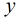与一些影响因素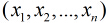之间关系的一种多变量分析方法。通常的问题是，研究某些因素条件下某个结果是否发生，比如医学中根据病人的一些症状来判断它是否患有某种病。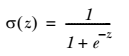### 基于最优化方法的回归系数确定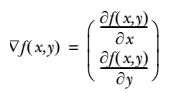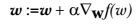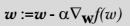Logistic回归 代价函数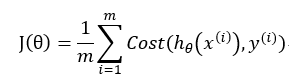，其中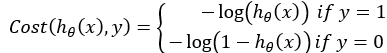hΘ(x)Cost(hΘ(x),y)之间的关系如下图：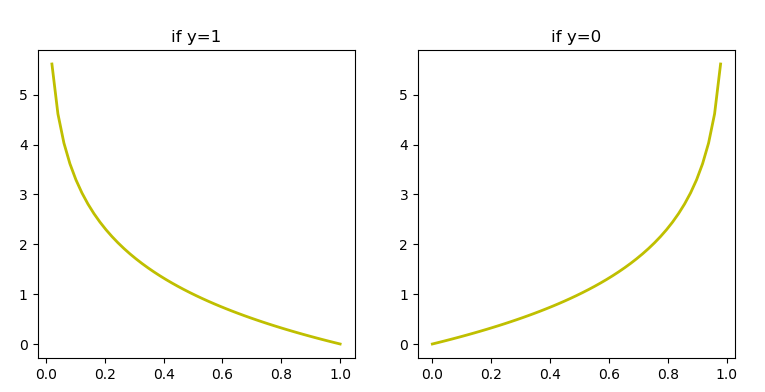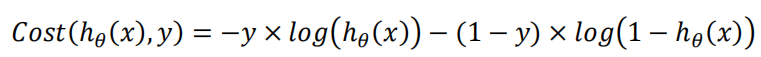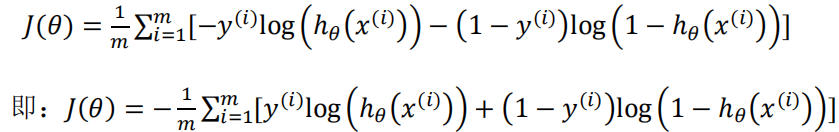Logistic回归 工作原理

计算整个数据集的梯度

使用 步长*梯度 更新回归系数向量

Logistic回归 算法特点

http://blog.csdn.net/achuo/article/details/51160101

项目案例

``````-0.017612	14.053064	0
-1.395634	4.662541	1
-0.752157	6.538620	0
-1.322371	7.152853	0
0.423363	11.054677	0``````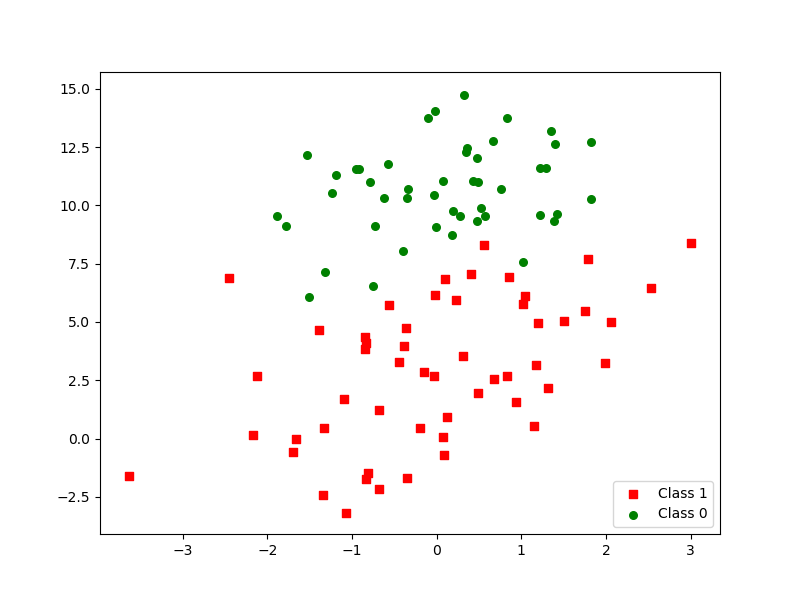代码实现

``````from math import exp
from numpy import *
from matplotlib import pyplot as plt
from matplotlib.font_manager import FontProperties

dataMat = []
labelMat = []
f = open(filename)
lineArr = line.strip().split()
dataMat.append([1.0, float(lineArr), float(lineArr)])
labelMat.append(int(lineArr))
return dataMat, labelMat

def sigmoid(inX):
return 1.0/(1+exp(-inX))

'''
梯度上升算法
:param dataMatIn: 数据集，一个二维Numpy数组，每列分别代表每个不同的特征，每行则代表每个训练样本。
:param classLabels: 类别标签，是一个（1，100）的行向量。
:return: 返回回归系数
'''
dataMatrix = mat(dataMatIn)
labelMat = mat(classLabels).transpose()
# m->样本数，n->特征数 在本例中m=100,n=3
m, n = shape(dataMatrix)
# alpha代表向目标移动的步长
alpha = 0.001
# 迭代次数
maxCycles = 500
# 权重值初始全为1
weights = ones((n, 1))
for k in range(maxCycles):
h = sigmoid(dataMatrix * weights)  # shape = (100,1)
error = labelMat - h  # shape = (100,1)
weights += alpha * dataMatrix.transpose() * error
return weights

'''
随机梯度上升法
:param dataMatIn: 数据集，一个二维Numpy数组，每列分别代表每个不同的特征，每行则代表每个训练样本。
:param classLabels: 类别标签，是一个（1，100）的行向量。
:param numIter: 外循环次数
:return: 返回回归系数
'''
dataMatrix = mat(dataMatIn)
m, n = shape(dataMatrix)
weights = ones((n, 1))
# 随机梯度, 循环150,观察是否收敛
for j in range(numIter):
# [0, 1, 2 .. m-1]
dataIndex = list(range(m))
for i in range(m):
# i和j的不断增大，导致alpha的值不断减少，但是不为0
alpha = 4/(1.0+j+i)+0.0001    # alpha 会随着迭代不断减小，但永远不会减小到0，因为后边还有一个常数项0.0001
# 随机产生一个 0～len(dataIndex)之间的一个值
# random.uniform(x, y) 方法将随机生成下一个实数，它在[x,y]范围内,x是这个范围内的最小值，y是这个范围内的最大值。
randIndex = int(random.uniform(0, len(dataIndex)))
choose = dataIndex[randIndex]
# sum(dataMatrix[i]*weights)为了求 f(x)的值， f(x)=w1*x1+w2*x2+..+wn*xn
h = sigmoid(sum(dataMatrix[choose]*weights))
error = classLabels[choose] - h
weights += alpha * error * dataMatrix[choose].transpose()
del(dataIndex[randIndex])
return weights

def showData(dataArr, labelMat):
'''
展示数据集分布情况
:param dataArr:
:param labelMat:
:return: None
'''
n = shape(dataArr)
xcord1 = []
ycord1 = []
xcord2 = []
ycord2 = []
for i in range(n):
if int(labelMat[i]) == 1:
xcord1.append(dataArr[i, 1])
ycord1.append(dataArr[i, 2])
else:
xcord2.append(dataArr[i, 1])
ycord2.append(dataArr[i, 2])
fig = plt.figure(figsize=(8, 6))
p1 = ax.scatter(xcord1, ycord1, s=30, c='red', marker='s')
p2 = ax.scatter(xcord2, ycord2, s=30, c='green')
plt.legend([p1, p2], ['Class 1', 'Class 0'], loc='lower right', scatterpoints=1)
plt.show()

def plotBestFit(dataArr, labelMat, weights1, weights2):
'''
将我们得到的数据可视化展示出来
:param dataArr:样本数据的特征
:param labelMat:样本数据的类别标签，即目标变量
:param weights:回归系数
:return:None
'''
font = FontProperties(fname=r"c:\windows\fonts\simsun.ttc", size=14)
n = shape(dataArr)
xcord1 = []
ycord1 = []
xcord2 = []
ycord2 = []
for i in range(n):
if int(labelMat[i]) == 1:
xcord1.append(dataArr[i, 1])
ycord1.append(dataArr[i, 2])
else:
xcord2.append(dataArr[i, 1])
ycord2.append(dataArr[i, 2])
fig = plt.figure(figsize=(8, 6))
ax1.scatter(xcord1, ycord1, s=30, c='red', marker='s')
ax1.scatter(xcord2, ycord2, s=30, c='green')
ax2.scatter(xcord1, ycord1, s=30, c='red', marker='s')
ax2.scatter(xcord2, ycord2, s=30, c='green')
x1 = arange(-3.0, 3.0, 0.1)
y1 = (-weights1 - weights1 * x1) / weights1
x2 = arange(-3.0, 3.0, 0.1)
y2 = (-weights2 - weights2 * x2) / weights2
ax1.plot(x1, y1)
ax2.plot(x2, y2)
ax1_title_text = ax1.set_title(u'梯度上升算法', FontProperties=font)
ax2_title_text = ax2.set_title(u'随机梯度上升算法', FontProperties=font)
plt.xlabel('X')
plt.ylabel('Y')
#plt.setp(ax1_title_text, size=20, weight='bold', color='black')
#plt.setp(ax2_title_text, size=20, weight='bold', color='black')
plt.show()

def testLR():
dataArr = array(dataMat)
test(dataArr, classLabels)
plotBestFit(dataArr, classLabels, weights1, weights2)

if __name__ == '__main__':
testLR()``````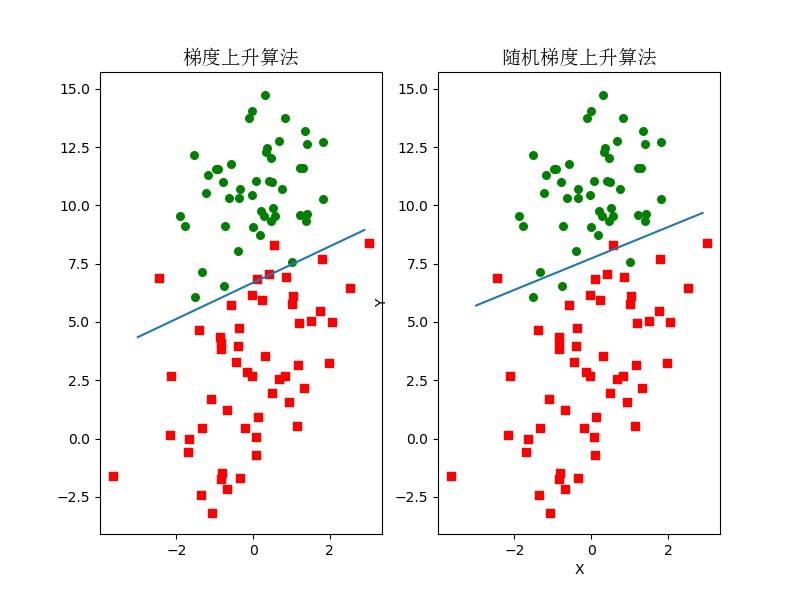o

### osc_g8254g7s

Logistic回归实战篇之预测病马死亡率(二)

06/30
0
0
2018文章集合

2018年公众号文章集合，过年在家系统学习下。 机器学习实战 该系列讲解了经典机器学习算法的原理（KNN，决策树，SVM，k-means，pca等），并从伪代码入手，一步步深入到各种算法的Python实现。...

2019/02/07
0
0
【机器学习】实战系列

SoWhat1412
04/19
1
0

qq_42154484
03/31
0
0
spark机器学习之常见的分类算法应用

on2way
06/23
0
0

linux下java环境搭建

wc_飞豆
38分钟前
17
0

40分钟前
22
0

zhixin9001
40分钟前
7
0

http://www.lookdiv.com/

40分钟前
27
0

1 前言 Helm是优秀的基于Kubernetes的包管理器。利用Helm，可以快速安装常用的Kubernetes应用，可以针对同一个应用快速部署多套环境，还可以实现运维人员与开发人员的职责分离。现在让我们安...

42分钟前
25
0